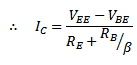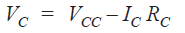# Transistor Biasing

## Transistor Biasing

In order to understand transistor biasing , you will have to understand faithful amplification first .The process of raising the strength of a weak signal without changing its shape is known as faithful amplification .

For faithful amplification, a transistor must satisfy the following conditions :

1. Proper zero signal collector current.
2. Minimum proper base-emitter voltage (VBE) at any instant.
3. Minimum proper collector-emitter voltage (VCE) at any instant.

### 1. Proper Zero Signal Collector Current

Let us consider an npn transistor circuit as shown in the figure below.During the positive half-cycle of the applied signal, base-emitter junction is forward biased and hence base current as well as large collector current flows in the circuit. Hence the positive half-cycle of the signal is amplified.

During the negative half-cycle, the base-emitter junction is reverse biased and hence no current flows in the circuit. Therefore, there is no output during the negative half-cycle of the signal.

Thus we will get an amplified output of the signal with no negative half cycles, which is unfaithful amplification.

Now let us introduce a battery source VBB in the base circuit as shown in the figure below.The magnitude of the battery should be such that, it keeps the input circuit forward biased for any value of the applied signal.

When  no signal is applied, a d.c. current IC flows in the collector circuit due to VBB, known as zero signal collector current.

During the positive half-cycle of the applied signal, input circuit is more forward biased and hence more collector current flows in the output circuit.

However, during the negative half-cycle, the input circuit is less forward biased and collector current decreases. Hence, the negative half-cycle also appears in the output.

Hence faithful amplification is achieved.

The zero signal collector current value should be at least equal to maximum collector current due to signal alone.

### 2. Proper Minimum Base-Emitter Voltage

For faithful amplification, the base-emitter voltage (VBE) should be larger than 0.5 V for Ge transistor and 0.7 V for Si transistor.

Since, the potential barrier for Ge is 0.5 V and for Si 0.7 V. Hence, In order to overcome this potential barrier, the base-emitter voltage should be larger than this voltage.

Once this potential barrier is overcome, the base current and hence the collector current increases sharply.

If the base-emitter voltage is less than this  value for any part of the applied signal, that part will be amplified lesser extent due to small collector current, which will result in unfaithful amplification.

### 3. Proper Minimum Collector-Emitter Voltage

For faithful amplification, the collector-emitter voltage should be larger than 0.5 V for Ge transistor and 1 V for si transistor. This is known as knee voltage.

When VCE  is less than this value , the collector-emitter junction is not properly reverse biased. Therefore, the collector can not attract the charge carriers emitter by the emitter, hence a grater portion of them goes to the base.

This causes a decrease in the collector current and increase in the base current. Hence, the applied signal will not be amplified properly and results in unfaithful amplification.

### Transistor Biasing

As we have already discussed, for faithful amplification, a transistor amplifier must satisfy the above three conditions. The fulfillment of these conditions is known as transistor biasing .

Transistor biasing can be defined as the proper flow of zero signal collector current and the maintenance of proper collector-emitter voltage during the passage of signal.

The basic purpose of transistor biasing is to keep the base-emitter junction forward biased and collector- base junction reverse biased at any instant of the applied signal.

### Methods of Transistor Biasing

The most commonly used methods for obtaining transistor biasing are:

1. Base resistor method
2. Emitter bias method
3. Biasing with collector-feedback resistor
4. Voltage divider bias

#### Base Resistor Method of Transistor Biasing

The circuit for base resistor biasing for an npn transistor is shown in the figure below.In this method, a high resistance Ris connected between the base and positive end of the supply voltage VCC.

Here, the required zero signal base current is provided by the supply voltage VCC , and it flows through resistance RB.

The required value of zero signal base current (IB) and hence the zeo signal collector current  I= βIB ,can be made to flow by selecting proper value of base resistor RB since the supply voltage VCC is fixed.

#### Circuit Analysis

It is required to find out the proper value of  Rso that required zero signal collector current flows in the collector circuit.

Let Ibe the required zero signal collector current.Now consider the closed circuit ABENA . Applying kirchoff ‘s voltage law:Since  value of VCC and Iare known and  VBE can be seen from the manual, hence value of Rcan be calculated from exp (i) .

1. This biasing circuit is very simple.
2. Biasing conditions can easily be set and calculations are simple.
3. There is no loading of the source by the biasing circuit since no resistor is connected across the base-emitter junction.

It has a poor stabilization, since there is no means to stop the self-increasing collector current due to temperature increase and individual variations.

#### Emitter Bias Method of Transistor Biasing

The circuit of  emitter  biasing for an npn transistor is shown in the figure below.This circuit differs from the base bias circuit in two respects, which are:

1. Here two supply voltages (VCC and VEE ) of equal value and opposite polarity are used instead of one supply voltage as in base bias circuit.
2. There is a resistor RE in the emitter circuit.

#### Circuit Analysis

From the above figure ,we will now find the Q-point values i.e. d.c. IC and d.c. VCE for this circuit.

#### (i) Collector Current (IC)

Applying Kirchhoff’s voltage law to the base-emitter circuit i.e. input circuit in the figure below, we get ,Now putting  I= IE/β in the above equation , we get#### (ii) Collector-emitter Voltage (VCE )

The figure below shows the various voltages of the emitter bias circuit with respect to ground.Emitter voltage w.r.t. ground is :Base voltage w.r.t. ground is :Collector voltage w.r.t. ground is :Now subtracting Vfrom Vand using the approximation I= IE, we get ,#### Transistor Biasing with Collector Feedback Resistor

In this method of biasing, one end of the resistor Ris connected to the base and the other end is connected to the collector as shown in the figure below .Here the required zero signal base current is determined by the collector-base voltage VCB, not by VCC .Since in this case VCB forward bias the base-emitter junction which causes the base current to flow trough RB which in result causes the zero signal collector current to flow in the circuit.

#### Circuit Analysis

The required value of Rneeded to give the zero signal collector current Ican be determined as follows :

From the figure above.Alternatively,#### Voltage Divider Bias Method of Transistor Biasing

This is the most widely used method of providing biasing to a transistor.

In this method of biasing , two resistances Rand R2 , connected across the supply voltage VCC  provide the required biasing as shown in the figure below.The emitter resistance Rprovides stabilization.

It is called voltage divider biasing due to the voltage divider formed by Rand R2,which provides biasing to the circuit.

The voltage drop across the resistance Rforward bias the base-emitter junction which causes the base current and hence the collector current to flow in the output circuit in zero signal condition.

#### Circuit Analysis

Let I1 be the flowing through the resistance R.

Since the base current in very small, therefore it can be assumed that current flowing through Ris also I1.

#### Collector Current (IC )Hence, voltage across Ris :Applying Kirchhoff’s voltage law to the base circuit, we getOr,Or,Since  IE≈ IC,From the above expression of IC , it is clear that it does not depends upon β. Though it depends upon VBE, but in practice is Vvery large as compared to VBE, hence practically IC is independent of VBE.

Therefore, Iis almost  independent of transistor parameters and hence it has a good stabilization.

#### Collector Emitter Voltage (VCE)

Applying Kirchhoff’s voltage law to the collector side of the fig.6 :